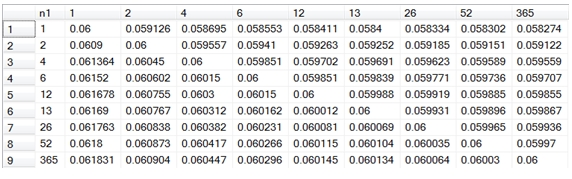# SQL Server equivalent rate function

PERIODRATE

Updated: 11 May 2012

Use PERIODRATE to adjust the nominal rate for a loan or other financial instrument when the compounding period of the quoted rate and the compounding period for the calculation of the loan are different. For example, if you wanted to turn a semi-annually compounded rate into its equivalent monthly-compounded rate.
Syntax
SELECT .[wct].[PERIODRATE] (
<@Nominal, float,>
,<@ComPeriod, float,>
,<@PayPeriod, float,>)
Arguments
@PV
the nominal or stated rate of interest. @Nominal is an expression of type float or of a type that can be implicitly converted to float.
@ComPeriod
the compounding period for @Nominal. @ComPeriod is an expression of type float or of a type that can be implicitly converted to float.
@PayPeriod
the compounding period the @Nominal is to be converted into. @PayPeriod is an expression of type float or of a type that can be implicitly converted to float.
Return Type
float
Remarks
·         @ComPeriod must be greater than or equal to 1
·         @PayPeriod must be greater than or equal to 1
·         @Payperiod must be greater than @ComPeriod
Example
Given a 7.0% semi-annually compounded rate, convert it into a monthly-compounded rate.
SELECT wct.PERIODRATE(
.07        --nominal rate of interest
,2          --semi-annual compounding
,12         --monthly compounding
) as [Monthly Rate]
This produces the following result.
Monthly Rate
----------------------
0.0690004739713013

In this example, we look at a variety of different compounding period combinations.
SELECT n1
,ROUND(, 6) as 
,ROUND(, 6) as 
,ROUND(, 6) as 
,ROUND(, 6) as 
,ROUND(, 6) as 
,ROUND(, 6) as 
,ROUND(, 6) as 
,ROUND(, 6) as 
,ROUND(, 6) as 
FROM (
SELECT n1.f as n1
,n2.f as n2
,wct.PERIODRATE(.06,n1.f,n2.f) as r1
FROM (
SELECT 1 UNION ALL
SELECT 2 UNION ALL
SELECT 4 UNION ALL
SELECT 6 UNION ALL
SELECT 12 UNION ALL
SELECT 13 UNION ALL
SELECT 26 UNION ALL
SELECT 52 UNION ALL
SELECT 365
) n1(f)
CROSS APPLY (
SELECT 1 UNION ALL
SELECT 2 UNION ALL
SELECT 4 UNION ALL
SELECT 6 UNION ALL
SELECT 12 UNION ALL
SELECT 13 UNION ALL
SELECT 26 UNION ALL
SELECT 52 UNION ALL
SELECT 365
) n2(f)
) d
PIVOT (MIN(r1) for n2 in (,,,,,,,,)
) as P
This produces the following result.If you look at the first row, it shows the result of 6.0% nominal annual rate converted to an equivalent semi-annual compounded rate, a quarterly compounded rate, a bi-monthly compounded rate, etc.
If you look at the first column is shows a 6.0% rate compounded annually, semi-annually, quarterly, bi-monthly, monthly, etc.

### SupportCopyright 2008-2023 Westclintech LLC         Privacy Policy        Terms of Service